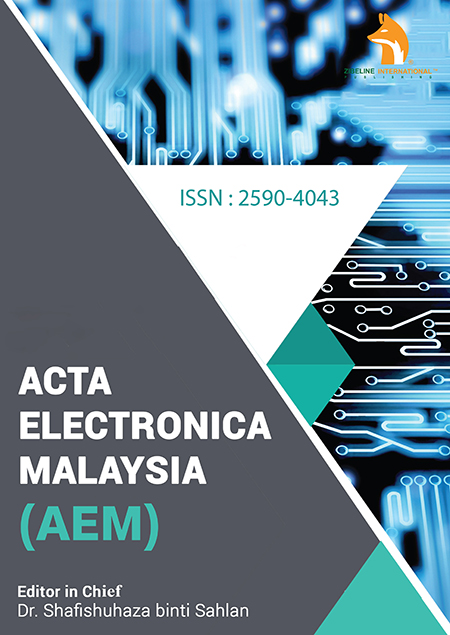# Acta Electronica Malaysia (AEM)

### ANALYSIS OF PLC-BASED AUTOMATED DOCK DOOR APPLICATION IN VEHICLE QUEUING SYSTEM OF COLD CHAIN

December 22, 2021 Posted by dgnoraina In Acta Electronica Malaysia (AEM)

## ABSTRACT

### INAUGURATION OF NEGATIVE POWER OF – N OF KIFILIDEEN TRINOMIAL THEOREM USING STANDARDIZED AND MATRIX METHODS

Acta Electronica Malaysia (AEM)
Author: Kifilideen L. Osanyinpeju

This is an open access article distributed under the Creative Commons Attribution License CC BY 4.0, which permits unrestricted use, distribution, and reproduction in any medium, provided the original work is properly cited

DOI :10.26480/aem.01.2021.17.23The positive power of Kifilideen trinomial theorem based on matrix and standardized approach had been developed and implemented alongside the general power combination formula which helps to determine the terms in the kifilideen expansion of positive power of n of trinomial expression. This study inaugurated negative power of − n of kifilideen trinomial theorem using standardized and matrix methods. Matrix was used in this research work to arrange the terms of the series of the negative power of the Kifilideen trinomial theorem. The general formula of the power combination of any term in the series was invented. The general formula to determine the term of a given power combination was also originated. It has been proving that the theorem and formulas generated are accurate, reliable, easy and interesting. The theorem helps in generating the terms of Kifilideen trinomial theorem of negative power of – n in an orderly form and makes it easy in obtaining the power combination that produce any given term and vice versa.

 Pages 17-23 Year 2021 Issue 1 Volume 5